## Taking pre-algebra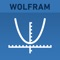# Wolfram Pre-Algebra Course Assistant

by Wolfram Group LLC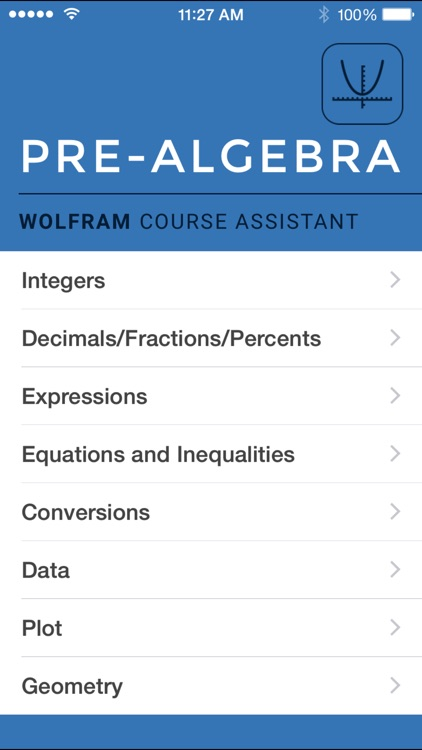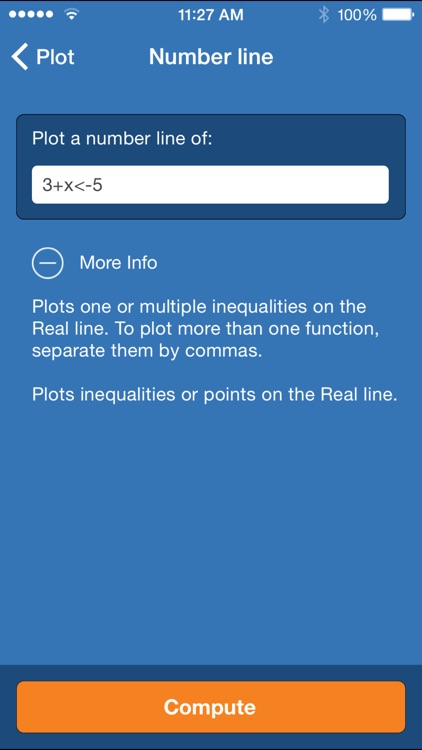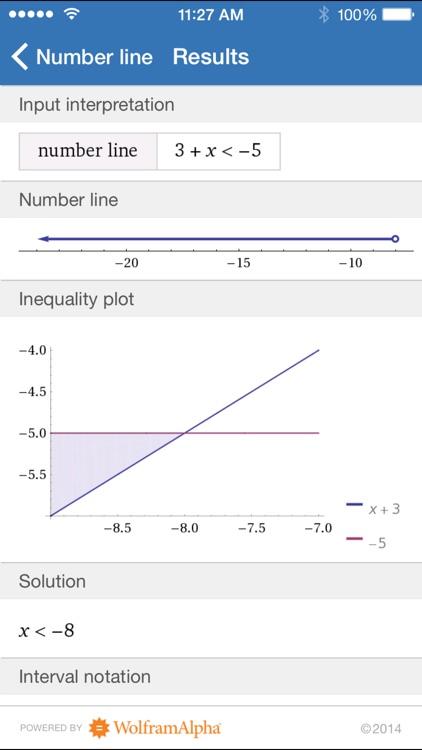Taking pre-algebra? Then you need the Wolfram Pre-Algebra Course Assistant. This definitive app for pre-algebra—from the world leader in math software—will help you work through your homework problems, ace your tests, and learn pre-algebra concepts. Forget canned examples! The Wolfram Pre-Algebra Course Assistant solves your specific pre-algebra problems on the fly.### App Details

Version
1.3.1
Rating
(1)
Size
12Mb
Genre
Education Reference
Last updated
June 1, 2017
Release date
August 3, 2011

### App Screenshots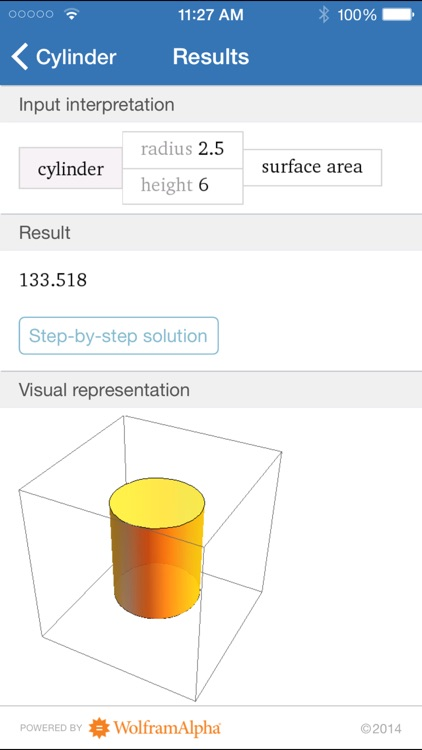### App Store Description

Taking pre-algebra? Then you need the Wolfram Pre-Algebra Course Assistant. This definitive app for pre-algebra—from the world leader in math software—will help you work through your homework problems, ace your tests, and learn pre-algebra concepts. Forget canned examples! The Wolfram Pre-Algebra Course Assistant solves your specific pre-algebra problems on the fly.

This app covers the following pre-algebra topics:

- Find the divisors and prime factorization of a number
- Calculate the GCD and LCM of two numbers
- Determine the percent change
- Reduce and round numbers
- Evaluate expressions
- Solve equations and simplify expressions
- Convert units of length, area, volume, and weight
- Compute the mean, median, and mode of a dataset
- Plot equations on the coordinate plane
- Graph inequalities on a number line
- Calculate the area, surface area, or volume of a geometric figure
- Find the midpoint, slope, and distance between two points

The Wolfram Pre-Algebra Course Assistant is powered by the Wolfram|Alpha computational knowledge engine and is created by Wolfram Research, makers of Mathematica—the world's leading software system for mathematical research and education.

The Wolfram Pre-Algebra Course Assistant draws on the computational power of Wolfram|Alpha's supercomputers over a 3G, 4G, or Wi-Fi connection.

Disclaimer:
AppAdvice does not own this application and only provides images and links contained in the iTunes Search API, to help our users find the best apps to download. If you are the developer of this app and would like your information removed, please send a request to takedown@appadvice.com and your information will be removed.